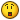# Date Calculator

## Recommended Posts

Hi all,

Here is my GUI:

```#include <GUIConstants.au3>
; == GUI generated with Koda ==
GUICreate("2Date", 250, 318)
\$MonthCal1 = GUICtrlCreateMonthCal("", 16, 40, 217, 193)
GUICtrlCreateLabel("Please select your birthdate in the below calender", 8, 8, 238, 17)
GUICtrlCreateLabel("You are:", 16, 270, 44, 17)
\$Input1 = GUICtrlCreateInput("", 16, 288, 217, 21, \$WS_EX_WINDOWEDGE & \$ES_CENTER)

\$Calc = GUICtrlCreateButton("Calculate", 72, 248, 113, 25, 0)
GUISetState(@SW_SHOW)
While 1
\$msg = GuiGetMsg()
Select
Case \$msg = \$GUI_EVENT_CLOSE
ExitLoop

Case \$msg = \$Calc
GUICtrlSetData(\$Input1, " days old ")

Case Else
;;;;;;;
EndSelect
WEnd
Exit```

What I am wanting is to calculate the number of days to date using the expression "year/month/day". For example : 20 years 2 months and 15 days.

I didn't see a similar reference in the forum so I googled and came across Microsoft's website where I got the info mentioned below. But quite honestly, I don't know how to integrate/convert this into an Autoit code. Can somebody assist please. The details from MS website are as below:

To calculate the number of days, months, and years between two dates, where the start and end dates are entered in cells A1 and A2 respectively, follow these steps:

1. Create a new workbook

2. Type the following data in the workbook (or any date you prefer):

A1: 10/01/94

A2: 05/03/06

3. Type the following formula in cell D1: =YEAR(A2)-YEAR(A1)-IF(OR(MONTH(A2)<MONTH(A1),AND(MONTH(A2)=MONTH(A1),

DAY(A2)<DAY(A1))),1,0)&" years, "&MONTH(A2)-MONTH(A1)+IF(AND(MONTH(A2)

<=MONTH(A1),DAY(A2)<DAY(A1)),11,IF(AND(MONTH(A2)<MONTH(A1),DAY(A2)

>=DAY(A1)),12,IF(AND(MONTH(A2)>MONTH(A1),DAY(A2)<DAY(A1)),-1)))&" months,

"&A2-DATE(YEAR(A2),MONTH(A2)-IF(DAY(A2)<DAY(A1),1,0),DAY(A1))&" days"

NOTE: If you copy and paste this formula, make sure that there are no line breaks, or the formula will not work.

If you typed the formula correctly, cell D1 now displays: 12 years, 1 months, 23 days.

Anyone know of a function that already does this or know how to use the above example in Autoit?##### Share on other sites

In date.au3 there are several date functions. _DateDiff is what you're looking for.

From the helpfile:

Returns the difference between 2 dates, expressed in the type requested.

#include <Date.au3>

_DateDiff(\$sType, \$sStartDate, \$sEndDate)

Parameters

\$sType:

D = Difference in days between the given dates

M = Difference in months between the given dates

Y = Difference in years between the given dates

w = Difference in Weeks between the given dates

h = Difference in hours between the given dates

n = Difference in minutes between the given dates

s = Difference in seconds between the given dates

\$sStartDate:

Input date in the format "YYYY/MM/DD[ HH:MM:SS]"

\$sEndDate:

Input End date in the format "YYYY/MM/DD[ HH:MM:SS]"

Return Value

Success: Difference between the 2 dates.

Failure: 0

@Error: 0 = No error.

1 = Invalid \$sType

2 = Invalid \$sStartDate

3 = Invalid \$sEndDate

##### Share on other sites

@greenmachine,

I did read the help file, but didn't find what I need. Perhaps my request wasn't understood clearly. I want a combination of the three: year + month + day.

```#include <Date.au3>

\$iDateCalc = _DateDiff( 'Y',"1994/01/10 00:00:00",_NowCalc())
MsgBox( 4096, "", "Number of years: " & \$iDateCalc )```

In the above code I get the result as 12 years. But in reality the precise figure (and the one I want) is supposed to be "12 years, 1 months, 23 days". Perhaps there is a way to mix and match the code in the help file, but I can't figure that out. Can someone assist please##### Share on other sites

Took me a while to get this idea. I was trying to do it manually, and it was tough.

```\$OldDate = "1994/01/10"
\$OldDateSplit = StringSplit (\$OldDate, "/")
\$iYearCalc = _DateDiff( 'Y', \$OldDate, _NowCalcDate())
\$OldDateSplit += \$iYearCalc
\$iMonthCalc = _DateDiff( 'M', \$OldDateSplit & "/" & \$OldDateSplit & "/" & \$OldDateSplit, _NowCalcDate())
\$OldDateSplit += \$iMonthCalc
If \$OldDateSplit > 12 Then
\$OldDateSplit += 1
\$OldDateSplit -= 12
EndIf
\$iDayCalc = _DateDiff( 'D', \$OldDateSplit & "/" & \$OldDateSplit & "/" & \$OldDateSplit, _NowCalcDate())
MsgBox (0, "date change", \$iYearCalc & @CRLF & \$iMonthCalc & @CRLF & \$iDayCalc)```
##### Share on other sites

Try This:

```\$StartDate = "1994/01/10 00:00:00"
\$EndDate = StringLeft(_NowCalc(),8) & StringMid(\$StartDate,9,10)
\$Years = _DateDiff("y", \$StartDate, \$EndDate)
\$Months = _DateDiff("m", \$StartDate, \$EndDate)
\$Months -= \$Years * 12
\$Days = _DateDiff("d", \$EndDate, _NowCalc())
if \$Days < 0 Then
\$Days += _DateDaysInMonth(StringLeft(\$EndDate,4), StringMid(\$EndDate,6,2) -1)
\$Months -= 1
EndIf
MsgBox(0, "Date Diff Calc", \$Years & " Year(s), " & \$Months & " Month(s), " & \$Days & " Day(s)")```
##### Share on other sites

@greenmachine & @GioVit,

Thank you very much for your assistance. Both your codes work. I was going crazy trying to come up with a solution, but you guys have solved it for me. Very much appreciate it.##### Share on other sites
• 16 years later...

I've written this piece of coding, with "no use of own calculations" but only "function calls".

```\$BegDate = "1994/01/10"
\$EndDate = _NowCalcDate()
\$Years   = _DateDiff("Y", \$BegDate, \$EndDate)
\$TmpDate = _DateAdd ("Y", \$Years  , \$BegDate)
\$Months  = _DateDiff("M", \$TmpDate, \$EndDate)
\$TmpDate = _DateAdd ("M", \$Months , \$TmpDate)
\$Days    = _DateDiff("D", \$TmpDate, \$EndDate)
MsgBox(0, "Date Diff Calc", \$Years & " Year(s), " & \$Months & " Month(s), " & \$Days & " Day(s)")```

## Create an account

Register a new account

• ### Recently Browsing   0 members

×

• Wiki

• Back

• #### Beta

• Git
• FAQ
×
• Create New...